# Prioritizr Quick Start Guide

## Introduction

This vignette is intended to provide a general walk-through of the functions available in the prioritizr package and their usage. Users should be familiar with the basic terminology and principles of systematic conservation prioritization. Please refer to the Prioritizr Basics vignette for more on the concepts behind the prioritizr package.

## Installation

To install the developmental version of prioritizr, use the following R code:

if (!require(devtools))
install.packages("devtools")
devtools::install_github("prioritizr/prioritizr")

## Overview

This package largely consists of seven main types of functions. These functions are used to:

• create a new reserve design problem by specifying the planning units and features of conservation interest (eg. species, ecosystems).
• add an objective to a reserve design problem.
• add targets to a problem to identify how much of each feature is desired in solutions
• add constraints to a problem to obtain better solutions.
• add penalties to a problem to penalize ineffective solutions.
• add decisions to a problem to specify the nature of the conservation decision on the planning units.
• add methods to generate a portfolio of solutions.
• solve a conservation problem.

The currently supported solvers are listed below. Each must be installed separately from this package. The details of the solvers are intentionally abstracted away so that minimal knowledge is required to use a given solver. However, one of the following R packages must be installed before a conservation problem can be solved.

## Work flow

The basic work flow for the prioritizer package starts with the creation of a problem, which specifies the user-defined input data on planning units, features, and costs. This new problem object can then be formulated by adding targets and objectives. All problems require an objective, which specifies the fundamental kind of conservation prioritization approach that will be used. Most problems require the addition of representation targets for input features as well, with the exception of the maximum cover problem (i.e. if the add_max_cover_objective function has been used), which seeks to maximize the features in the solution and therefore does not require targets for individual features.

Depending on the needs of the user, constraints and penalties can be added next. This includes the options to lock in or lock out particular planning units, or penalize solutions with low connectivity (similar to the boundary length modifier used in Marxan). Alternative decision formats can also be specified using the decisions functions, otherwise the package will default to binary selection of planning units. Lastly, the type of solving algorithm must be specified by installing one of the supported solving packages and adding the solver to the problem using one of the solvers functions.

A problem object is solved using the solve function, which will return a raster-class object, spatial-class object, or a numeric vector containing the solution depending on the object class initially provided in problem formulation. Additional parameters can be created and customized according to the specific needs of the user.

We will work through the problem formulation process with a simple example that uses one of the built-in simulated data sets that is distributed within the package. First, load the prioritizr package.

# load prioritizr package
library(prioritizr)
library(rasterVis)
library(viridis)
library(ggplot2)

### Problem Formulation

A reserve design exercise starts by determining the spatial extent of the planning units in your study area. The problem function is used to specify the basic data used in a spatial prioritization problem: the spatial distribution of the planning units and their costs, as well as the features (eg. species, ecosystems) that need to be conserved. Prioritizr supports a range of input data formats including rasters, shapefiles, tables, and arrays. Note that the type of input provided to the package for the planning units will also be the type of data file returned in the solution.

The general usage of the problem function is p <- problem(x, features, ...)

Where x is the input for planning units, features are the biodiversity features to be used, and ... are additional arguments depending on the input to x.

In this simulated example, biodiversity features are represented using a stack of raster data (ie. RasterStack objects). A RasterStack represents a collection of RasterLayers with the same spatial properties (ie. spatial extent, coordinate system, dimensionality, and resolution). Each RasterLayer in the stack describes the distribution of a biodiversity feature.

In our example, the sim_features object is a RasterStack object that contains raster::nlayers(sim_features) layers. Each RasterLayer describes the distribution of a species. Specifically, the cell values denote the proportion of suitable habitat in across the study area. For a given layer, cells with a value of one are entirely comprized of suitable habitat for the feature, and cells with a value of zero contain no suitable habitat.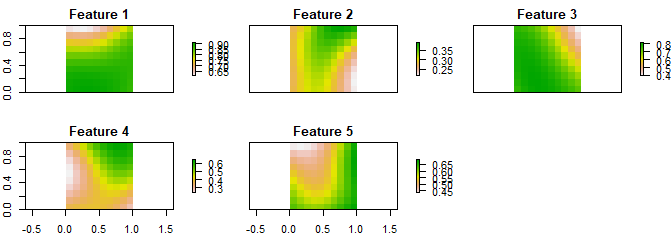We use a simulated dataset in this tutorial, but to use your own data with prioritizr, you first need to prepare your dataset. This is the most important part of the prioritizr workflow, and where most errors are likely to arise. If the solve function doesn’t run correctly, the first thing to check should be that your data are not malformed and comply with the problem formulation specifications.

The formulation of different data input types is detailed below.

#### Shapefile Input

If the planning unit data is contained in a shapefile, R will convert this into spatial-class object, where attribute data can be called from a data frame. Prioritizr recognizes point, line, or polygon inputs. The spatial-class problem object is more flexible than the problem formulated for raster inputs, because planning units can be irregularly shaped and cost data can be incorporated from spatial, raster, and data frame sources. However, spatial-class planning units can take longer to process than raster planning units when constraints or penalties are added to problems that require information on spatial proximity or adjacency (e.g. add_boundary_penalties), so raster planning units are recommended where possible. Note that, all else being equal, there is no difference on the time spent solving a problem that has spatial-class or raster planning units. The example dataset we use here contains the option to use any of the three spatial-class inputs.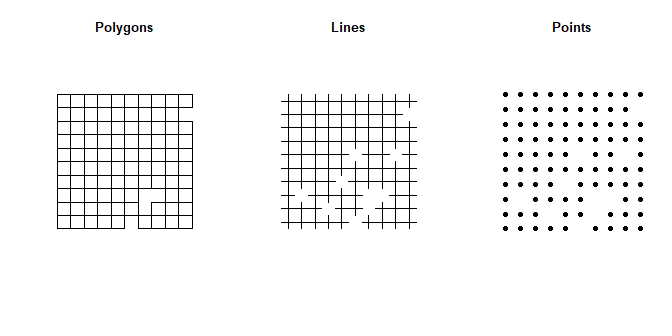In this example dataset, all of the simulated spatial-class objects contain the same attributes, with cost as the first column and additional information on the status of each planning unit as the subsequent columns.

# explore simulated data
head(sim_pu_polygons)
##       cost locked_in locked_out
## 1 215.8638     FALSE      FALSE
## 2 212.7823     FALSE      FALSE
## 3 207.4962     FALSE      FALSE
## 4 208.9322     FALSE       TRUE
## 5 214.0419     FALSE      FALSE
## 6 213.7636     FALSE      FALSE

To create a conservation problem object to which you can add targets, constraints, etc., use the problem function with the spatial-class planning unit object and the features object as arguments. In this example we use the sim_features raster stack to represent features, but you can also use a spatial-class object where the distributions of multiple features are included as attribute columns. Unless the problem being formulated exactly matches Marxan formatting (see the Marxan problem section in this tutorial), cost must be explicitly defined using the cost_column argument. Cost can be an attribute of the planning unit spatial-class object, or derived from a separate raster, data.frame, or numeric vector aligned with planning unit ID. If the cost column is in the planning unit spatial object, you will have to identify the cost column within the attribute table.

# polygons
p1 <- problem(sim_pu_polygons, sim_features, cost_column = "cost")
# lines
p2 <- problem(sim_pu_lines, sim_features, cost_column = "cost")

# points
p3 <- problem(sim_pu_points, sim_features, cost_column = "cost")

#### Raster Input

If the planning unit data input is a raster, then the argument to x should be a single-band raster where each pixel is a planning unit containing the corresponding cost information. For example, in the sim_pu_raster object, the cell value denotes the simulated cost of acquiring that pixel. Raster-class problem objects have the advantage of solving more efficiently than spatial-class problem objects, but planning units must be regularly shaped, which is not always feasible.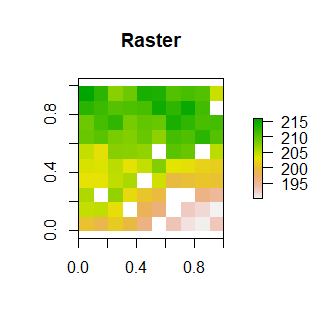The argument to features must be a raster-class object with the same spatial properties as the planning unit raster (i.e. spatial extent, coordinate system, dimensionality, and resolution). In this example, we again use the sim_features object. To create a conservation problem class object, use the same notation as with spatial-class input. Note that the cost data can not be included as a separate argument to the problem function in this case.

# raster planning units
p4 <- problem(sim_pu_raster, sim_features)

#### Data Frame or Numeric Vector Input

Planning unit data can also be uploaded from a .csv file into a data.frame, or entered into a numeric vector. The argument to x should contain one row for each planning unit with its corresponding cost. The argument to features must also be a data.frame or matrix, following the conventions used by Marxan. Each row corresponds to a different feature, and must contain the following columns:

• "id": Unique identifier for each feature.
• "name": Name for each feature.
• "prop": Relative target for each feature (optional). Targets can also be added using the targets functions.
• "amount": Absolute target for each feature (optional).

Because data.frame and numeric vector input formats do not include spatial data, you will need to include a table specifying the quantity of each feature in each planning unit. This is called a representation matrix or RIJ matrix, and is identified in the problem function by the argument rij (data.frame) or rij_matrix (numeric vector). For data.frame inputs, the argument to rij must follow the conventions used by Marxan. It must contain the following columns:

• "pu": Planning unit identifier.
• "species": Feature identifier matching the ID used in the argument to features.
• "amount": Amount of the feature in the planning unit.

For numeric vector inputs, the argument to rij_matrix can be a simple Matrix-class object specifying the amount of each feature (rows) within each planning unit (columns).

Because the formatting for data frame and numeric vector problem objects follows the Marxan input file specifications, users may wish to use the marxan_problem function instead of the problem function; which allows users to quickly and easily solve Marxan problems with a single function. Although this method does not provide as many customizations, it may be more convenient for users already familiar with Marxan problems or with existing Marxan data files. For more details see the __Marxan_ Problem_ section at the end of this tutorial.

Note: If the input to problem are spatial data such as a raster or a spatial-class object, the RIJ matrix is calculated automatically.

Once the problem is formulated, the next step is to add an objective. A problem objective is used to specify the overall goal of the problem. All conservation planning problems involve minimizing or maximizing some kind of objective. For instance, the planner may require a solution that conserves enough habitat for each species while minimizing the overall cost of the reserve network. Alternatively, the planner may require a solution that maximizes the number of conserved species while ensuring that the cost of the reserve network does not exceed the budget. Objectives are added in the same way regardless of the type of data input to problem. For simplicity, the examples from this point onward will only use the problem formulated for the simulated raster dataset.

The prioritizr package contains four objective functions: * add_min_set_objective: Used to find a solution that fulfills all the targets and constraints for the smallest cost. This objective is similar to that used in Marxan. For example, we can add a minimum set objective to our simulated raster problem as follows:

# create new problem object from scratch
p4 <- problem(sim_pu_raster, sim_features) %>%

# create new problem object based on an existing problem object:
p4 <- problem(sim_pu_raster, sim_features)
p5 <- p4 %>% add_min_set_objective()
• add_max_cover_objective: Used to represent one instance of as many features as possible within a given budget. We can add a maximum cover objective to our simulated problem using the same notation as for the minimum set objective, but with the addition of a user-specified budget.
p4 <- problem(sim_pu_raster, sim_features) %>%
add_max_cover_objective(budget = 5000)
• add_max_features_objective: Used to find a solution that fulfills as many targets as possible while ensuring that the cost of the solution does not exceed budget and that all constraints are met. This objective was inspired by the conservation problem defined in Cabeza and Moilanen (2001). Like the maximum cover objective, a budget must be specified as an argument to the maximum features objective function.
p4 <- problem(sim_pu_raster, sim_features) %>%
add_max_features_objective(budget = 5000)
• add_max_phylo_objective: Used to find a solution that fulfills as much of a representative sample a phylogenetic tree as possible given a budget. This objective is similar to the maximum features objective except that emphasis is placed on phylogenetic representation rather than target representation. This objective requires the ape R package to be installed. In the simulated dataset contained in the prioritizr package there is the sample phylogenetic data object sim_phylogeny.
data(sim_phylogeny) #load simulated phylogeny data
p4 <- problem(sim_pu_raster, sim_features) %>%
add_max_phylo_objective(budget = 5000, tree = sim_phylogeny)
• add_max_utility_objective: Used to find a solution that secures as much of each feature as possible without exceeding the budget.
p4 <- problem(sim_pu_raster, sim_features) %>%
add_max_utility_objective(budget = 5000)

The %>% notation is necessary to tell the package which problem to attach the objective to. Note that when multiple objectives are added to the same problem object, only the most recent objective is used. For details on the mathematical formulation of each type of objective function, please refer to the Prioritizr Basics vignette.

Most conservation planning problems require the addition of targets. Targets are used to specify the minimum amount or proportion of a feature’s distribution that needs to be protected. For example, we may want to develop a reserve network that will secure 20% of the distribution for each feature for minimal cost. There are three types of targets available in prioritizr: relative targets, where targets are set as a proportion (between 0 and 1) of the maximum level of representation of features in the study area; absolute targets, where targets are expressed as the actual value of features in the study area that need to be represented; and log-linear targets, where targets are expressed as a proportion (between 0 and 1) and calculated using a log-linear equation and four tuning parameters related to range size. This last target type is based on the approach used in Rodrigues et al. (2004).

We can add targets to our simulated problem object in the same way that objectives are added. The %>% notation tells the package which problem to attach the objectives and targets to.

# add relative targets to existing problem
p4 <- problem(sim_pu_raster, sim_features) %>%

# Or, create new problem objects to try different target types
# create problem with added relative targets

# create problem with added absolute targets

# create problem with added log-linear target
p.l <- p4 %>% add_loglinear_targets(10, 0.9, 100, 0.2)

Targets can be a single value applied to all features, or you can use a vector of values corresponding to each feature. For example, you may wish to place a greater emphasis on Feature 1 than the other features contained in sim_features.

# vary targets by feature
p.r2 <- p4 %>% add_relative_targets(c(0.5, 0.1, 0.1, 0.1, 0.1))

As with the objective funtions, when multiple targets are added to the same problem object, only the most recent target setting is used. If a maximum cover objective is used, then targets do not need to be set, as all features will be maximized.

A constraint can be added to a conservation planning problem as a way to make certain solutions invalid, given a cutoff criteria. They are used when specific planning units or configurations of planning units are undesireable or inefficient. The following constraint functions are available in the prioritizr package:

• add_connected_constraints: Add constraints to a conservation problem to ensure that all selected planning units are spatially connected to each other.
• add_corridor_constraints: It is important to maintain connectivity between reserves. However, some areas are more difficult for species to traverse then other areas. As a consequence, even though reserves may be connected, species may not be able to move between reserves if the areas connecting them are barriers to dispersal. This function adds constraints to ensure that corridors connect reserves and that individuals from all species can utilize the corridors. Friction raster-class objects are used to identify difficult areas to traverse.
• add_locked_in_constraints: Add constraints to ensure that they are prioritized in the solution. For example, it may be desirable to lock in planning units that are inside existing protected areas so that the solution fills in the gaps in the existing reserve network.
• add_locked_out_constraints: Add constraints to ensure that certain planning units are not prioritized in the solution. For example, it may be useful to lock out planning units that have been degraded and are not longer suitable for conserving species.
• add_neighbor_constraints: Add constraints to a conservation problem to ensure that all selected planning units have at least a certain number of neighbors.

Add constraints to a conservation problem in the same way that targets and objectives are added.

# add relative targets to existing problem
p4 <- problem(sim_pu_raster, sim_features) %>%
add_connected_constraints()
The add_locked_in_constraints and add_locked_out_constraints are useful for masking particular planning units in or out. There are several ways to identify planning units for masking. In our simulated dataset, the sim_pu_polygons Spatial-class object contains a locked_in and locked_out attribute to identify which planning units should always be included in solutions or never included in solutions, respectively. Alternatively, you may wish to use a separate raster layer as a mask, such as the sim_locked_in_raster or sim_locked_out_raster contained in the simulated dataset.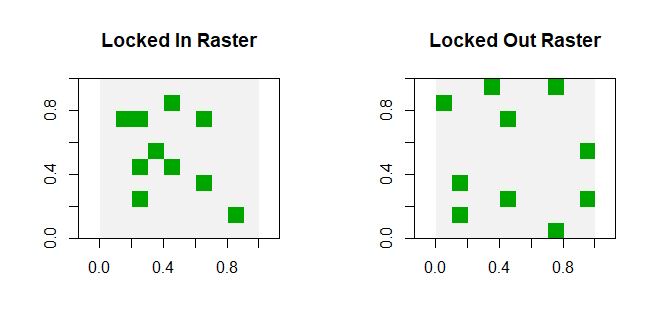# create problem with added locked in constraints using integers
p.lock1 <- p1 %>% add_locked_in_constraints(which(sim_pu_polygons$locked_in)) # create problem with added locked in constraints using a field name p.lock2 <- p1 %>% add_locked_in_constraints("locked_in") # create problem with added locked in constraints using raster data p.lock3 <- p4 %>% add_locked_in_constraints(sim_locked_in_raster) ### Add Penalties A highly fragmented solution may be associated with increased management costs and be susceptible to edge effects. A penalty can be applied to a conservation planning problem to penalize solutions with low connectivity between planning units. This acts as an explicit trade-off with the objective being minimized or maximized, and thereby increases the cost of the solution. For example, when the a boundary length penalty is added (equivalent to a boundary length modifier in Marxan), solution fragmentation decreases but solution cost increases. There are two types of penalty available in prioritizr: • add_boundary_penalties: Add penalties to a conservation problem to favor solutions that clump selected planning units together into contiguous reserves. Uses shared boundary length as a measure of connectivity, equivalent to the boundary length modifier (BLM) in Marxan. Boundary data is calculated automatically unless the planning units in the problem object are stored in a data.frame, in which case boundary data must be explicitly added as a matrix or data.frame. This function can only be used for symmetric relationships between planning units. • add_connectivity_penalties: Add constraints to a conservation problem to favor solutions that select planning units with high connectivity between them. Uses connectivity data in the form of a matrix or data.frame object, where strength of connectivity is a value such as the inverse distance between consecutive planning units. This function can be used for symmetric or asymmetric relationships between planning units. For example, we can apply a boundary penalty to our simulate problem using a high penalty factor of 500 (ie. boundary length modifier; BLM), and an edge factor of 50% so that planning units along the coastline are not overly penalized. # create problem with boundary penalties added to it pBLM <- p4 %>% add_boundary_penalties(penalty = 500, edge_factor = 0.5) A penalty can be applied to a problem that already has one or more constraints added, but users are cautioned that overly restrictive constraints and penalties can lead to a solving error. For example, the following solutions are for three minimum set problems, with targets set to 20% for all features, using binary decision making and a Gurobi solver. The solutions differ only by the constraints or penalties added. p_1 <- problem(sim_pu_raster, sim_features) %>% add_min_set_objective() %>% add_relative_targets(0.2) %>% add_gurobi_solver() p_2 <- problem(sim_pu_raster, sim_features) %>% add_min_set_objective() %>% add_relative_targets(0.2) %>% add_boundary_penalties(penalty = 500, edge_factor = 1) %>% add_gurobi_solver() # solve problems s <- stack(solve(p_1), solve(p_2)) # plot solutions plot(s, main = c("basic solution", "boundary penalties"))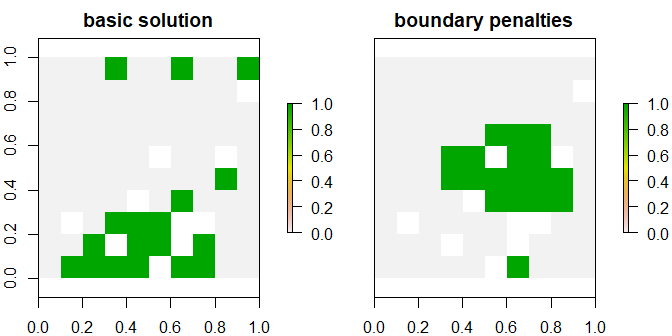### Specify the type of decision variable Conservation planning problems involve making decisions on planning units. These decisions are then associated with actions (eg. turning a planning unit into a protected area). If no decision is explicitly added to a problem, then the binary decision class will be used by default. This applies to scenarios where you can either purchase all of the land inside a given planning unit or none of the land inside a given planning unit. If it is desireable to acquire part of a planning unit, you can use the proportion decision option or the semicontinuous decision option. The following decision classes are available in prioritizr: • add_binary_decisions: Add a binary decision to a conservation planning problem. This is the classic decision of either prioritizing or not prioritizing a planning unit. Typically, this decision has the assumed action of buying the planning unit to include in a protected area network. If no decision is added to a problem object then this decision class will be used by default. • add_proportion_decisions: Add a proportion decision to a problem. This is a relaxed decision where a part of a planning unit can be prioritized, as opposed to the default of the entire planning unit. Typically, this this decision has the assumed action of buying a fraction of a planning unit to include in a protected area network. • add_semicontinuous_decisions: Add a semi-continuous decision to a problem. This decision is similar to proportion decisions except that it has an upper bound parameter. By default, the decision can range from prioritizing none (0%) to all (100%) of a planning unit. However, a upper bound can be specified to ensure that at most only a fraction (eg. 80%) of a planning unit can be preserved. This type of decision may be useful when it is not practical to conserve the entire planning unit. ### Solve a problem Finally, a problem object that is formulated to your specifications can be solved to obtain a solution. If you do not specify the type of solver used, prioritizr will automatically use the best solver currently installed. We recommend installing the Gurobi software suite and the gurobi R package to solve problems quickly. If Gurobi is not an option, install one of the SYMPHONY solvers. The functions used to add a specific solver are: • add_gurobi_solver: Gurobi is a state-of-the-art commercial optimization software with an R package interface. It is by far the fastest of the solvers available in this package, however, it is also the only solver that is not freely available. That said, licenses are available to academics at no cost. The gurobi package is distributed with the Gurobi software suite. • add_rsymphony_solver: SYMPHONY is an open-source integer programming solver that is part of the Computational Infrastructure for Operations Research (COIN-OR) project, an initiative to promote development of open-source tools for operations research (a field that includes linear programming). The Rsymphony package provides an interface to COIN-OR and is available on CRAN. • add_lpsymphony_solver: The lpsymphony package provides a different interface to the COIN-OR software suite. Unlike the Rsymhpony package, the lpsymphony} package is distributed through Bioconductor. On Windows and Mac, lpsymphony may be easier to install. Use the solve function to produce a solution. # formulate the problem p5 <- problem(sim_pu_raster, sim_features) %>% add_min_set_objective() %>% add_relative_targets(0.1) %>% add_boundary_penalties(penalty = 500, edge_factor = 0.5) %>% add_binary_decisions() %>% add_gurobi_solver() # solve the problem s5 <- solve(p5) plot(s5, col = c("grey90", "darkgreen"), main = "Solution", xlim = c(-0.1, 1.1), ylim = c(-0.1, 1.1))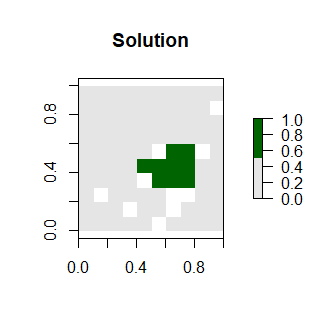We can plot this solution because the planning unit input data are spatially linked. The output format will always match the planning unit data input in problem formulation. For example, the solution for a problem with planning units in a shapefile would be a spatial-class object with an attribute indicating if each planning unit was selected in the solution. If the planning units were in a data.frame, the solution would be a table indicating whether each planning unit was selected or not. The solve function automatically compresses and compiles the problem from a ConservationProblem-class object into an OptimizationProblem-class object, which is a series of matrices and tables specifically designed for integer linear programming algorithms to work with. The compile function can be used to manually compress a problem object into an Optimization problem, but this is not recommended unless the user is familiar with the formatting of the Optimization problem and wishes to manipulate it directly. The solve function intentionally abstracts away the optimization process for simplicity. We can also extract extra information from the solution. This information shows the solution’s objective value, the time spent solving the problem, and a message describing why the solution was returned (e.g. because the optimal solution was found? Because a time limit was exceeded?). # extract the objective (cost of solution in this case) print(attr(s5, "objective")) ## solution_1 ## 2366.175 # extract time spent solving solution print(attr(s5, "runtime")) ## solution_1 ## 0.2041607 # extract message returned from solver print(attr(s5, "status")) ## solution_1 ## "OPTIMAL" ### Additional customizations If the functions available in prioritizr do not meet the specific needs of a project it is possible to create parameters using the parameters functions. Only experts should work directly with these functions. ## The Marxan problem Although users are encouraged to build and tailor conservation planning problems to suit their own needs using the problem function, sometimes it is easier to use a more familiar “canned” approach. The _Marxan_problem function provides a convenient wrapper for generating and solving Marxan-style conservation problems. If users already have their conservation planning data in the Marxan input format, this function can also be used to read Marxan data files and solve the Marxan-style problems using exact algorithm solvers. With the marxan_problem function, targets, boundary length penalties, and locked in/out constraints are included as arguments in the initial problem formulation, rather than added with separate functions. The options available are limited to those that would be available with Marxan - users wishing for greater flexibility should use the problem function instead. For example, problems formulated with marxan_problem are always minimum set problems. A Marxan problem is solved in the same way as a problem object, and therefore still requires the installation of one of the solver packages. prioritizr contains Marxan input files that can be used as an example dataset. # complete input file input <- system.file("extdata/input.dat", package = "prioritizr") mp1 <- marxan_problem(input) ms1 <- solve(mp1) head(ms1) ## id cost status xloc yloc locked_in locked_out solution_1 ## 1 3 0.0 0 1116623 -4493479 FALSE FALSE 0 ## 2 30 752727.5 0 1110623 -4496943 FALSE FALSE 0 ## 3 56 3734907.5 0 1092623 -4500408 FALSE FALSE 0 ## 4 58 1695902.1 0 1116623 -4500408 FALSE FALSE 0 ## 5 84 3422025.6 0 1098623 -4503872 FALSE FALSE 0 ## 6 85 17890758.4 0 1110623 -4503872 FALSE FALSE 0 Marxan input files can also be supplied individually. pu <- system.file("extdata/input/pu.dat", package = "prioritizr") %>% read.table(sep = ",", header = TRUE) features <- system.file("extdata/input/spec.dat", package = "prioritizr") %>% read.table(sep = ",", header = TRUE) bound <- system.file("extdata/input/bound.dat", package = "prioritizr") %>% read.table(sep = "\t", header = TRUE) rij <- system.file("extdata/input/puvspr.dat", package = "prioritizr") %>% read.table(sep = ",", header = TRUE) mp2 <- marxan_problem(x = pu, spec = features, puvspr = rij, bound = bound, blm = 0) The marxan_problem function can also read non-Marxan input files such as spatial and raster layers, provided they are formatted following the conventions used by Marxan input files. Problem formulation differs slightly with this method. Targets can be either relative or absolute, and planning units can be specified for masking in or out using the locked in and locked out arguments. Connectivity can be added with the penalty argument, which imposes a penalty on solutions with high boundary lengths (equivalent to the Boundary Length Modifier (BLM) used in Marxan), and the edge_factor argument to scale the penalty for edges that do not have neighboring planning units, such as coastline. Cost does not have to be explicitly identified with marxan_problem, because if column names follow Marxan conventions, the cost column can be located by the function. The simulated dataset we use here can be read as a Marxan problem. A problem with no boundary penalty, but a locked in constraint, would be formulated as follows. mp3 <- marxan_problem(x = sim_pu_polygons, features = sim_features, targets = 0.2, targets_type = "relative", locked_in = sim_locked_in_raster, cost_column = "cost") ms3 <- solve(mp3) spplot(ms3, zcol = "solution_1", main = "Solution")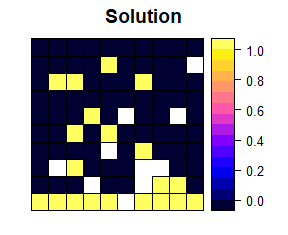## Post-Processing Solutions There are several options for post-processing solutions if you’re interested in conducting additional analyses beyond plotting. Post-processing can be done efficiently by exporting solutions into GIS software platforms such as ArcGIS and QGIS, but it is also possible to calculate statistics on spatial layers within R. Some handy functions for raster solutions include zonal and extract from the raster package; zonal when the features are rasters, and extract when the features are spatial layers. The cellStats and layerStats functions from the raster package are also useful. For solutions from Marxan input files, post-processing can be done as if it were a data.frame. # count number of planning units used to represent each feature zonal(s5, sim_features[], fun = sum) # feature 1 ## zone value ## [1,] 1 9 zonal(s5, sim_features[], fun = sum) # feature 2 ## zone value ## [1,] 0 9 zonal(s5, sim_features[], fun = sum) # feature 3 ## zone value ## [1,] 0 0 ## [2,] 1 9 zonal(s5, sim_features[], fun = sum) # feature 4 ## zone value ## [1,] 0 7 ## [2,] 1 2 zonal(s5, sim_features[], fun = sum) # feature 5 ## zone value ## [1,] 0 0 ## [2,] 1 9 # count number of selected planning units sum(ms1$solution_1 == 1)
##  482